# SAT Math : How to find the length of the side of an acute / obtuse triangle

## Example Questions

← Previous 1

### Example Question #112 : Triangles

A triangle has sides of length 8, 13, and L. Which of the following cannot equal L?

6

7

9

15

4

4

Explanation:

The sum of the lengths of two sides of a triangle cannot be less than the length of the third side. 8 + 4 = 12, which is less than 13.

### Example Question #2 : How To Find The Length Of The Side Of An Acute / Obtuse Triangle

Two sides of a triangle are 20 and 32.  Which of the following CANNOT be the third side of this triangle.

13

15

10

17

20

10

Explanation:

Please remember the Triangle Inequality Theorem, which states that the sum of any two sides of a triangle must be greater than the third side.  Therefore, the correct answer is 10 because the sum of 10 and 20 would not be greater than the third side 32.

### Example Question #3 : How To Find The Length Of The Side Of An Acute / Obtuse Triangle

A triangle has sides of length 5, 7, and x. Which of the following can NOT be a value of x?

13

7

5

3

11

13

Explanation:

The sum of the lengths of any two sides of a triangle must exceed the length of the third side; therefore, 5+7 > x, which cannot happen if x = 13.

### Example Question #4 : How To Find The Length Of The Side Of An Acute / Obtuse Triangle

The lengths of two sides of a triangle are 9 and 7. Which of the following could be the length of the third side?

1

16

17

2

12

12

Explanation:

Let us call the third side x. According to the Triangle Inequality Theorem, the sum of any two sides of a triangle must be larger than the other two sides. Thus, all of the following must be true:

x + 7 > 9

x + 9 > 7

7 + 9 > x

We can solve these three inequalities to determine the possible values of x.

x + 7 > 9

Subtract 7 from both sides.

x > 2

Now, we can look at x + 9 > 7. Subtracting 9 from both sides, we obtain

x > –2

Finally, 7 + 9 > x, which means that 16 > x.

Therefore, x must be greater than 2, greater than –2, but also less than 16. The only number that satisfies all of these requirements is 12.

### Example Question #5 : How To Find The Length Of The Side Of An Acute / Obtuse Triangle

The lengths of a triangle are 8, 12, and x. Which of the following inequalities shows all of the possible values of x?

4 < x <12

8 < x < 12

4 ≤ x ≤12

4 < x < 20

4 ≤ x ≤ 20

4 < x < 20

Explanation:

According to the Triangle Inequality Theorem, the sum of any two sides of a triangle must be greater (not greater than or equal) than the remaining side. Thus, the following inequalities must all be true:

x + 8 > 12

x + 12 > 8

8 + 12 > x

Let's solve each inequality.

x + 8 > 12

Subtract 8 from both sides.

x > 4

Next, let's look at the inequality x + 12 > 8

x + 12 > 8

Subtract 12 from both sides.

x > –4

Lastly, 8 + 12 > x, which means that x < 20.

This means that x must be less than twenty, but greater than 4 and greater than –4. Since any number greater than 4 is also greater than –4, we can exclude the inequality x > –4.

To summarize, x must be greater than 4 and less than 20. We can write this as 4 < x < 20.

The answer is 4 < x < 20.

### Example Question #6 : How To Find The Length Of The Side Of An Acute / Obtuse Triangle

If 2 sides of the triangle are have lengths equal to 8 and 14, what is one possible length of the third side?

22

6

Not enough information

4

20

20

Explanation:

The sum of the lengths of 2 sides of a triangle must be greater than—but not equal to—the length of the third side. Further, the third side must be longer than the difference between the greater and the lesser of the other two sides; therefore, 20 is the only possible answer.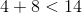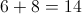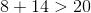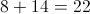### Example Question #561 : Geometry

In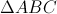the length of AB is 15 and the length of side AC is 5. What is the least possible integer length of side BC?

10

13

11

9

17

11

Explanation:

Rule - the length of one side of a triangle must be greater than the differnce and less than the sum of the lengths of the other two sides.

Given lengths of two of the sides of theare 15 and 5. The length of the third side must be greater than 15-5 or 10 and less than 15+5 or 20.

The question asks what is the least possible integer length of BC, which would be 11

### Example Question #1 : How To Find The Length Of The Side Of An Acute / Obtuse Triangle

Two sides of a triangle have lengths 4 and 7. Which of the following represents the set of all possible lengths of the third side, x?

4 < x < 7

2 < x < 12

3 < x < 7

3 < x < 11

4 < x < 11

3 < x < 11

Explanation:

The set of possible lengths is: 7-4 < x < 7+4, or 3 < X < 11.

### Example Question #9 : How To Find The Length Of The Side Of An Acute / Obtuse Triangle

If two sides of a triangle have lengths 8 and 10, what could the length of the third side NOT be?

14

19

10

5

8

19

Explanation:

According to the Triangle Inequality Theorem, the sums of the lengths of any two sides of a triangle must be greater than the length of the third side. Since 10 + 8 is 18, the only length out of the answer choices that is not possible is 19.

### Example Question #10 : How To Find The Length Of The Side Of An Acute / Obtuse Triangle

Given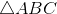with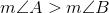and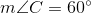.

Which of the following could be the correct ordering of the lengths of the sides of the triangle?

I)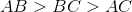II)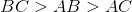III)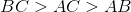I only.

II only.

II or III only.

I or II only.

III only.

II only.

Explanation:

Given two angles of unequal measure in a triangle, the side opposite the greater angle is longer than the side opposite the other angle. Therefore, we seek to find the relationship among the measures of the angles., and the measures of the interior angles of a triangle total, so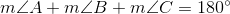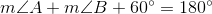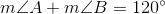Since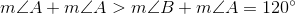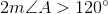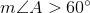and, similarly,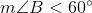Therefore,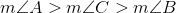and the lengths of their opposite sides rank similarly:.

The correct response is that only (II) can be true.

← Previous 1